#### You may also like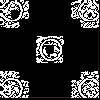### Doplication

We can arrange dots in a similar way to the 5 on a dice and they usually sit quite well into a rectangular shape. How many altogether in this 3 by 5? What happens for other sizes?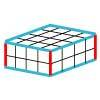### Making Cuboids

Let's say you can only use two different lengths - 2 units and 4 units. Using just these 2 lengths as the edges how many different cuboids can you make?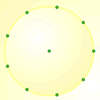How many DIFFERENT quadrilaterals can be made by joining the dots on the 8-point circle?

# Round and Round the Circle

## Round and Round the Circle

I started with a clock without hands or the minute divisions (except for those where there is a number). The $12$ was replaced by a $0$ and the numbers placed outside the face.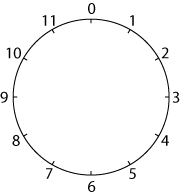I ruled lines joining up the numbers.

I started by counting in ones and I got a $12$-gon (that is a $12$-sided polygon - if you like long words you can call it a dodecagon).Then I ruled lines counting round in $2$s. And I got .....?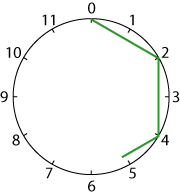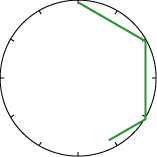Perhaps you do not need to put the numbers round the circles.

I tried $5$s (wow!) and $6$s (well!).

Each time I go on drawing lines until I get to the point where I first started.

Then I tried $7$s, $8$s, $9$s, $10$s, and $11$s.

Something interesting was happening.

Why don't you try it? What patterns do you notice emerging?

And what about counting round in $12$s?

Which shapes are the same? Can you think of a reason why?

Can you see a connection between the number in which you are counting around the circle and the number of sides in the shape you are making?

### Why do this problem?

This problem brings out patterns in numbers, factors and multiples as well as properties of shapes. There are plenty of opportunities for visualising to predict what patterns will emerge. Learners will need to work systematically and to generalise, and the work done also often makes a very appealing 'finished product' for display purposes.

### Possible approach

You could start with a clock with the numbers round the outside of the circle, but change the $12$ into a $0$ as in the question. If you are able to display the image on an interactive whiteboard, you will be able to draw round in ones and then start the twos (as in the question). Ask the group to predict what will happen, and then to check by continuing to draw on the board. Make sure that they understand that they go on round the circle until they reach $0$ again.

Many children have a problem with counting round in $5$s. To avoid this show them how it is done on the board. Start at $0$ count round $5$ and make a mark by the relevant place on the circle. Now join the $0$ to this mark. Again, count round $5$ and make a mark by the relevant place on the circle and join this to the place where the last line finished. Continue doing this until you reach $0$ again. You should have visited every point round the circle by this time.

Children can then work on the challenge in pairs - encourage them to make predictions before testing them. Make sure that they realise that every number should be done on a new dial. This sheet of six numbered circles, which makes a good starting point, can be printed out. Alternatively, this sheet has twelve copies of the $12$-circle.

After a suitable length of time, draw learners together to share their findings. You may want to collate their results on the board as pairs demonstrate the drawing or show their sketches. Ask them to explain how the shapes relate to the number of divisions round the circles, looking out for those who use appropriate vocabulary such as 'factor' and 'multiple'. Which numbers produced the same shapes? Ask again about the relationship of these to the number of divisions round the circles. Press the group to generalise their findings as this can bring out interesting facts about factors, multiples and primes. You might find this interactivity helpful in the discussion.

(If you want to make mathematical stars for Christmas or Divali make sheets with circles divided into a prime number of divisions such as $5$, $7$, $11$ and $13$.)

### Key questions

After doing the $12$s and $10$s, learners could try to predict which shapes will be produced with other numbers on the circle before testing their predictions. This sheet has three copies each of circles marked in $10$s, $9$s, $8$s and $7$s. You might be able to introduce the concept of 'co-prime'.
Most difficulties with this problem (at the start at least) lie in the actual drawing rather than in the numbers. Encourage learners to pencil very lightly so the work can be rubbed out if necessary and to mark where they are going before starting to draw the line. Pupils often have trouble with counting round in $5$s so it might help to go through the process, stage by stage: start at $0$, count round $5$ and make a mark by the relevant place on the circle. Now join the $0$ to this mark. Again, count round $5$ and make a mark by the relevant place on the circle and join this to the place where the last line finished. Continue doing this until $0$ is reached again. Every point round the circle should have been visited by this time.